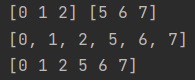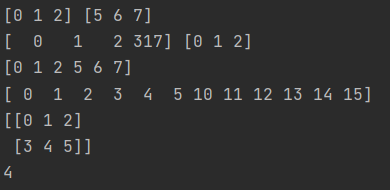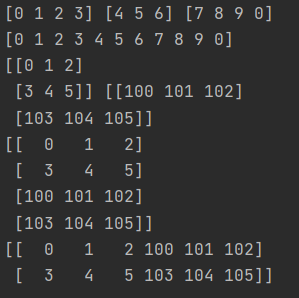• python两列数据拼接
2020-10-23 11:43:36
#1.merge
import pandas as pd
data=pd.merge(AA,BB,how='left',left_on="aa",right_on="bb")
data=pd.merge(AA,BB,how='left',on=["a1","a2"]) #同时用两列拼接
data=pd.merge(AA,BB,how='left',left_index=TRUE,right_index=FALSE)

#2.CONCAT
data=pd.concat([AA,BB],join='inner',axis=0,ignore_index=TRUE)#axis=0 表示上下合并，axis=1表示左右合并，默认为0上下合并，ignore_index=True 表示忽略之前的索引

#3.append 上下合并
data=AA.append([BB,CC],ignore_index=TRUE)python
更多相关内容
• 1.先将数组转换为列表，后用列表的拼接函数append()、extend()进行拼接，最后将列表转换为数组。 import numpy as np x=np.array([0,1,2]) y=np.array([5,6,7]) print(x,y) listx=list(x) listy=list(y) listx....

1.先将数组转换为列表，后用列表的拼接函数append()、extend()进行拼接，最后将列表转换为数组。

import numpy as np
x=np.array([0,1,2])
y=np.array([5,6,7])
print(x,y)
listx=list(x)
listy=list(y)
listx.extend(listy)
print(listx)
x=np.array(listx)
print(x)2.numpy库中有numpy.append(arr, values, axis=None)函数。对参数有一定的限制：数组和数值或者两个数组，
不能三个以上的数组进行直接的append函数拼接。

import numpy as np
x=np.array([0,1,2])
y=np.array([5,6,7])
print(x,y)
t=np.append(x,317)
print(t,x)
k=np.append(x,y)
print(k)
A=np.array([[0,1,2],[3,4,5]])
B=np.array([[10,11,12],[13,14,15]])
u=np.append(A,B)
print(u)
print(A)
print(A)#为什么此处u不对了我们注意到，虽然A、B是二维数组，但拼接后的u却是一维的，这是因为np.append(arr, values, axis=None)在其函数参数axis未指定时，会将arr和values（values可以是值，也可以是数组）降到一维再连接，所以u就会报错。

3.利用numpy.concatenate((a1,a2,…), axis=0)函数。能够一次完成多个数组的拼接。

import numpy as np
x=np.array([0,1,2,3])
y=np.array([4,5,6])
z=np.array([7,8,9,0])
k=np.concatenate((x,y,z),axis=0)
print(x,y,z)
print(k)
#axis=0不写则默认为0
#对一维数组的拼接，axis的值不影响结果
A=np.array([[0,1,2],[3,4,5]])
B=np.array([[100,101,102],[103,104,105]])
t=np.concatenate((A,B),axis=0)#axis=0表示直接进行拼接
print(A,B)
print(t)
p=np.concatenate((A,B),axis=1)
#axis=1表示对应行的数组进行拼接
print(p)展开全文python
• python用符号拼接DataFrame两列

# 问题描述

如下图的日期dataframe,需要把开始日期和结束日期拼接在一起

原dataframe

开始日期结束日期
2020-08-032020-08-09
2020-08-102020-08-16
2020-08-172020-08-23
2020-08-242020-08-30
2020-08-312020-09-06

拼接后的dataframe

开始日期结束日期插入日期
2020-08-032020-08-092020-08-03 ~ 2020-08-09
2020-08-102020-08-162020-08-10 ~ 2020-08-16
2020-08-172020-08-232020-08-17 ~ 2020-08-23
2020-08-242020-08-302020-08-24 ~ 2020-08-30
2020-08-312020-09-062020-08-31 ~ 2020-09-06

# 解决方案

## 方案一：apply映射

# 方案1
date_xl['插入日期']=date_xl.apply(lambda x:x['开始日期']+" ~ "+x['结束日期'],axis=1)

# 方案2
date_xl['插入日期']=date_xl.apply(lambda x:" ~ ".join(x.values),axis=1)



上面两种方法，原理基本一致

碰到Null值时，会报错，因为none不可与str运算
解决如下，加入if判断即可

df = pd.DataFrame([list("ABCDEF"),
list("ABCDE")]).T
df.columns=list('XY')
df.apply(lambda x:" ~ ".join(x.values) if (x.values!= None) &(x.values != None) else np.nan,axis=1)


## 方案二：转成嵌套数组/列表

# 转换成嵌套数组
df.values
np.array(df)

#转换成嵌套列表
df.values.tolist()
np.array(df).tolist()

# 拼接
pd.DataFrame([" ~ ".join(i)  if (i!= None) &(i != None) else np.nan for i in np.array(df).tolist()])

展开全文python
• python两列字符串如何合并？python两列字符串合并的方法：1、在很多情况下，我们都需要合并字符串。例如：需要将姓氏与名字存储在不同的变量中，然后显示的时候再将他们合二为一first_name = 'oliver'last_name = '...python两列字符串如何合并？

python两列字符串合并的方法：

1、在很多情况下，我们都需要合并字符串。例如：需要将姓氏与名字存储在不同的变量中，然后显示的时候再将他们合二为一first_name = 'oliver'

last_name = 'smith'

full_name = first_name + ' ' + last_name

print(full_name)

打印结果：

oliver smith

2、python中使用（+）号来合并字符串

这种字符串合并的方法称为拼接，可使用存储在变量中的信息来创建完整的信息first_name = 'oliver'

last_name = 'smith'

full_name = first_name + ' ' + last_name

print('Hello ' + full_name.title() + '!')

打印结果：

Hello Oliver Smith!

此处的title()方法将单词的首字母大写了，让姓名显示更格式化

3、也可以创建用一个变量来存储整条语句first_name = 'oliver'

last_name = 'smith'

full_name = first_name + ' ' + last_name

msg = 'Hello ' + full_name +'!'print(msg)

这样，print语句中显示的就没那么复杂了！

推荐教程：《python视频教程》

以上就是python两列字符串如何合并？的详细内容，更多请关注gxlcms其它相关文章！

本条技术文章来源于互联网，如果无意侵犯您的权益请点击此处反馈版权投诉

本文系统来源：php中文网

展开全文• Python 如何编写交互界面？_后端开发Python如何编写交互界面：首先导入Python GUI库tkinter；然后使用tkinter接口“Tk...python两列字符串合并的方法：1、在很多情况下，我们都需要合并字符串。例如：需要将姓氏与名...
• ## python将两列合并

千次阅读 2020-11-29 11:50:17
这个问题在这里已有答案：在pandas python 19答案的 数据框中组合两列文本 我想将多个列连接成一列。 我的列标签名为“comments”，“comment1”和“comment2”。 在每个列中，我希望将值连接在一起。 下面给出了我...
• ## python下的数据拼接

千次阅读 2020-12-06 14:19:36
使用pandas拼接1. pd.merge()1. pd.merge()1. pd.merge()功能：用于通过一个或多个键将两个数据集的行连接起来，类似于 SQL 中的 JOIN。语法如下：merge(left, right, how='inner', on=None, left_on=None, right_on...
• 我试图能够使用来自指定列的数据从pandas数据帧生成相关矩阵这是我的csv数据：col0,col1,col2,col3,col4122468.9071,1417464.203,3546600,151804924,1083947614691.1139,170036.0407,103847,19208604,2365065以下是...
• ## python合并两列

千次阅读 2020-11-30 09:12:59
将一份表格文件中不同 sheet 单页面中的数据汇总整理到一起 python 自动整理 excel表格 摘要：将两份表格通过共有的数据信息进行合并融合给无网络的办公电脑插上 python小翅膀摘要：windows 办公电脑在无网络情况下...
• python_datafram两列拼接，中间加上特殊字符 t10['temp'] = t10['date'].apply(lambda x: x+ ':') t10['temp'].head() t10['user_date_datereceived_gap'] = t10['temp'] + t10['date_received'] t10['user_date_...
• 主要介绍了Python实现自定义顺序、排列写入数据到Excel的方法,涉及Python针对Excel文件的数据处理及读写相关操作技巧,需要的朋友可以参考下
• 默认concat，参数axis=0, join=‘outer’, ignore_index=False，即按行拼接拼接方式保留所有列，不忽略原数据索引。 import pandas as pd pd.concat([df1, df2]) 返回结果 设置ignore_index=True，忽略原数据...python
• 我目前遇到两个问题：我的数据框架如下所示：, male_female, no_of_students0, 24 : 76, "81,120"1, 33 : 67, "12,270"2, 50 : 50, "10,120"3, 42 : 58, "5,120"4, 12 : 88, "2,200"我想要实现的是：, male, female,...
• python7 静态方法、类方法、属性方法 ；反射；异常处理 #-*- coding:utf8 -*- # 静态方法@staticmethod # 静态方法(当eat变成静态方法后,再通过实例调用时就不会自动把实例本身当作一个参数传给self了.) clas ... ...
• 我有一个问题，要在同一个数据帧(start_end)中将两列合并为一列，还要删除空值。我打算将“起始站”和“结束站”合并为“站”，并根据新列“站”保留“持续时间”。我试过pd.merge，pd.concat，pd.append，但是我没...
• python中如何实现列表元素内容合并不是加到后面而是 list1=[a,b,c] list2=[1,2,3] 如何实现，让 list3=['alist1=['a','b','c']list2=['1','2','3']n=len(list1)list3=[]for i in range(0,n):list3.append('')for i ...
• 需求：dataframe中两列需要合并成一列，字符串并用“—”连接 解决： df["ydate"] =df["y"].map(str) +"-"+ df["date"].map(str) 如果是两列数值相加，则直接两列相加即可 df["add"] =df["a"] + df["b"]python
• 我在Python中使用熊猫有20 x 4000数据帧。Two of these columns are named Year and quarter.这些列中的两个分别命名为Year和Quarter。I'd like to create a variable called period that makes Year = 2000 and ...
• try: file = "./" + file_name + ".XLS" df = pd.read_excel(file) # 读取excle文件 time_test = df['日期'].map(str) + " " + df['时间'].map(str) # 日期加时间拼接 df["new_time"] = pd.DataFrame(time_test) # ...python
• 如图操作 非常多行，且每一列的文字存在数字，各种标点 <p><img alt="Excel怎样合并两个列中的文本内容" src=...image%2Fresize%2Cm_lfit%2Cw_500%2Climit_1%2Fquality%2Cq_80" /></p>python
• Pandas包的merge、join、concat方法可以完成数据的合并和拼接，merge方法主要基于两个dataframe的共同列进行合并，join方法主要基于两个dataframe的索引进行合并，concat方法是对series或dataframe进行行拼接或列...
• 问题描述：在一个 220*2 的矩阵 A 中，以行为单位(即每行)，行与行之间不存在重复的数据。现已经得到了矩阵 A 中的 i 行数据构成的矩阵 B，求另外 220-i 行数据构成的矩阵 C 。...本文实验环境：Python 3....
•python
• 使用Python 处理csv文件时，想要的操作两列合并为一列，感觉比较简单，操作起来有点小麻烦 代码 import pandas as pd df = pd.read_csv("aaaa.csv") df["ls"] = df.iloc[:,1:3].values.tolist()python
• 怎么使用python编写一个能把列表内所有元素前面都创建python文件，文件名为：testlistadd.py；编写代码，在原有list的每个元素前面加上字符‘1’； old_list = ['a','b','c','d'] new_list = ['1'+x for x in old_...
• Python进行数据处理时，遇到点的坐标以x，y两列数据显示，而具体的操作时，又需要用（x，y）这种点的坐标的形式来进行筛选分析，针对这一问题，以下几行代码便轻松解决！ 首先先来读取数据。 import pandas as ...Python dataframe
• 问题描述 我有一个用于模型训练的DataFrame如下图所示： 其中的country、province、city、county四列其实是位置信息的不同层级，应该合成一列用于模型训练 ...如果某一列是非str类型的数据，那么我们需要用到map(s
• ## pandas 两列数据合并

千次阅读 2020-11-18 18:32:12
, 'quarter': ['q1', 'q2']}) df['year_quarter'] = df['year'] + "_" + df['quarter'] # 方法1 df['year|year'] = df[['year', 'quarter']].agg('|'.join, axis=1) # 方法2 # 主要注意的是 如果存在 nan 数据怎么办...
• 遇到的问题是两个字符串拼接，查到了三种对多列同时操作的方法，其中两种可用 生成列表，可直接填充为df中一列 df['new_col']=list(map(lambda x,y: function(x,y), df['col1'], df['col2'])) 参考见： ...python
• 目的：表亲+近亲+自己，统计孤身一人和非孤身一人的信息 for dataset in full_data: dataset['FamilySize'] = dataset['SibSp'] + dataset['Parch'] + 1 # 家庭规模：表亲+近亲+自己python...